Courses

# JEE Main Mathematics Mock - 4

## 25 Questions MCQ Test Mock Test Series for JEE Main & Advanced 2022 | JEE Main Mathematics Mock - 4

Description
This mock test of JEE Main Mathematics Mock - 4 for JEE helps you for every JEE entrance exam. This contains 25 Multiple Choice Questions for JEE JEE Main Mathematics Mock - 4 (mcq) to study with solutions a complete question bank. The solved questions answers in this JEE Main Mathematics Mock - 4 quiz give you a good mix of easy questions and tough questions. JEE students definitely take this JEE Main Mathematics Mock - 4 exercise for a better result in the exam. You can find other JEE Main Mathematics Mock - 4 extra questions, long questions & short questions for JEE on EduRev as well by searching above.
QUESTION: 1

Solution:
QUESTION: 2

Solution:
QUESTION: 3

### Let f(x) be a function satisfying f ′(x) = f (x) with f(0) = 1 and g(x) be a function that satisfies f(x) + g(x) = x2, then value of integral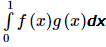is equal to

Solution:
QUESTION: 4

If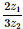is a purely imaginary number, then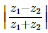is equal to

Solution:
QUESTION: 5

If f (x) = cos [π2] x + cos [− π2] x where [x] is the step function, then

Solution:
QUESTION: 6

The sum of the focal distances from any point on the ellipse 9x2 + 16y2 = 144 is

Solution:
QUESTION: 7

In the following question, a Statement of Assertion (A) is given followed by a corresponding Reason (R) just below it. Read the Statements carefully and mark the correct answer-
Assertion(A): f (x) = log x3 and g (x) = 3 log x are equal.
Reason(R) : Two functions f and g are said to be equal if their domains, ranges are equal and f (x) = g (x)∀ x in the domain .

Solution:
QUESTION: 8

Determinant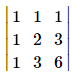is not equal to

Solution:
QUESTION: 9

If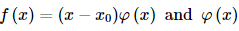is continuous at x = x0 , then f ′ (x0) is equal to

Solution:
QUESTION: 10

Solution of the differential equation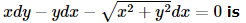Solution:
QUESTION: 11

In the following question, a Statement-1 is given followed by a corresponding Statement-2 just below it. Read the statements carefully and mark the correct answer-
Tangents are drawn from the point (17,7) to the circle x2+y2=169.
Statement-1:
The tangents are mutually perpendicular.
Statement-2:
The locus of the points from which mutually perpendicular tangents can be drawn to the given circle is x2+y2=338.

Solution:
QUESTION: 12

If z2 = -i, then z is equal o

Solution:
QUESTION: 13

The sum of the digits in the unit place of all the numbers formed with the help of 3,4,5,6 taken all at a time is

Solution:
If the unit place is ‘3’ then remaining three places can be filled in 3! ways.
Thus ‘3’ appears in unit place in 3! times.
Similarly each digit appear in unit place 3! times.
So,  sum of digits in unit place = 3!(3 + 4 + 5 + 6) = 18 * 6 = 108
QUESTION: 14

If θ + Φ = π/3 then sin θ . sinΦ has a maximum value at θ =

Solution:

Here, y = sin θ · sinΦ = sinθ · sin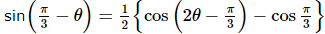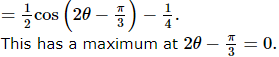QUESTION: 15

An unbiased coin is tossed to get 2 points for turning up a head and one point for the tail. If three unbiased coins are tossed simultaneously, then the probability of getting a total of odd number of points is

Solution:
QUESTION: 16

The probability of A = probability of B = probability of C = 1/4, P(A∩B) = P(C∩B) = 0 and P(A∩C) = 1/8, then P(A∪B∪C) is equal to:

Solution: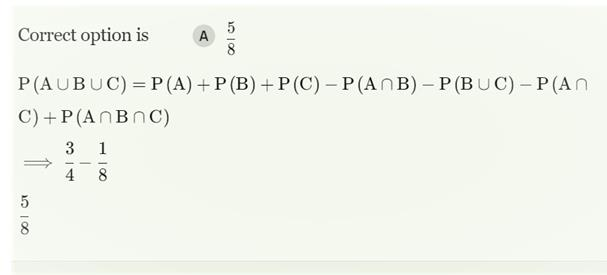QUESTION: 17

If the equations x2+ ba + a = 0 and x2 + ax + b = 0 have a common root, then a + b =

Solution:
QUESTION: 18

If a, b, c, d, e, f are in A.P., then e - c is equal to

Solution:
QUESTION: 19

If a line passes through points (4,3) and (2,λ) and perpendicular to y=2x+3, then λ=

Solution:
QUESTION: 20

The median of a set of 9 distinct observations is 20.5. If each of the largest 4 observations of the set is increased by 2, then the median of the new set

Solution:
*Answer can only contain numeric values
QUESTION: 21

If a, b are odd integers then number of integral root, of equation x10 + ax9 + b = 0 is equal to :

Solution:

Let P is a root (∈I)
Case-I : p is odd
p10 + ap9 + b ≠ 0
Because LHS odd
Case-II : p is even
p10 + ap9 + b ≠ 0
because, LHs is odd

*Answer can only contain numeric values
QUESTION: 22

If the number of distinct positive rational numbers p/q smaller than 1, where  p, q ∈ {1, 2, 3 ....., 6} is k then k is :

Solution:

Out of numbers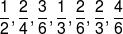will result only 3 distinct rational numbers.
⇒ Total numbers = 6C2 – 7 + 3 = 11

*Answer can only contain numeric values
QUESTION: 23

If two distinct chords of a parabola y2 = 4ax passing through (a, 2a) are bisected on the line x + y = 1, then the sum of integral values of the length of possible latus rectums is equal to :

Solution:

Any point on x + y = 1 can be taken as (t, 1–t)
The equation of chord with this as mid-point is y(1–t) –2a (x + t) = (1 – t)2 – 4at
It passes through (a, 2a)
So, t2 – 2t + 2a2 – 2a + 1 = 0
This should have two distinct real roots. So D > 0
⇒ a2 – a < 0
⇒ 0 < a < 1
So, length of latus rectum < 4 and 0 < a < 1
⇒ LR = 1, 2, 3

*Answer can only contain numeric values
QUESTION: 24

If  the value of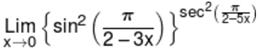is e–A then ‘A’ is :

Solution: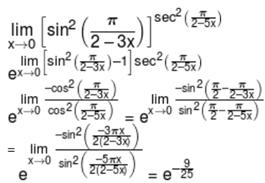*Answer can only contain numeric values
QUESTION: 25

If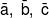be three vectors of magnitude √3, 1, 2, such that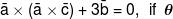is the angle between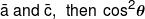is equal  to:-

Solution: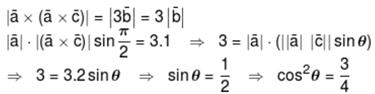Track your progress, build streaks, highlight & save important lessons and more!

### Similar Content### Related tests